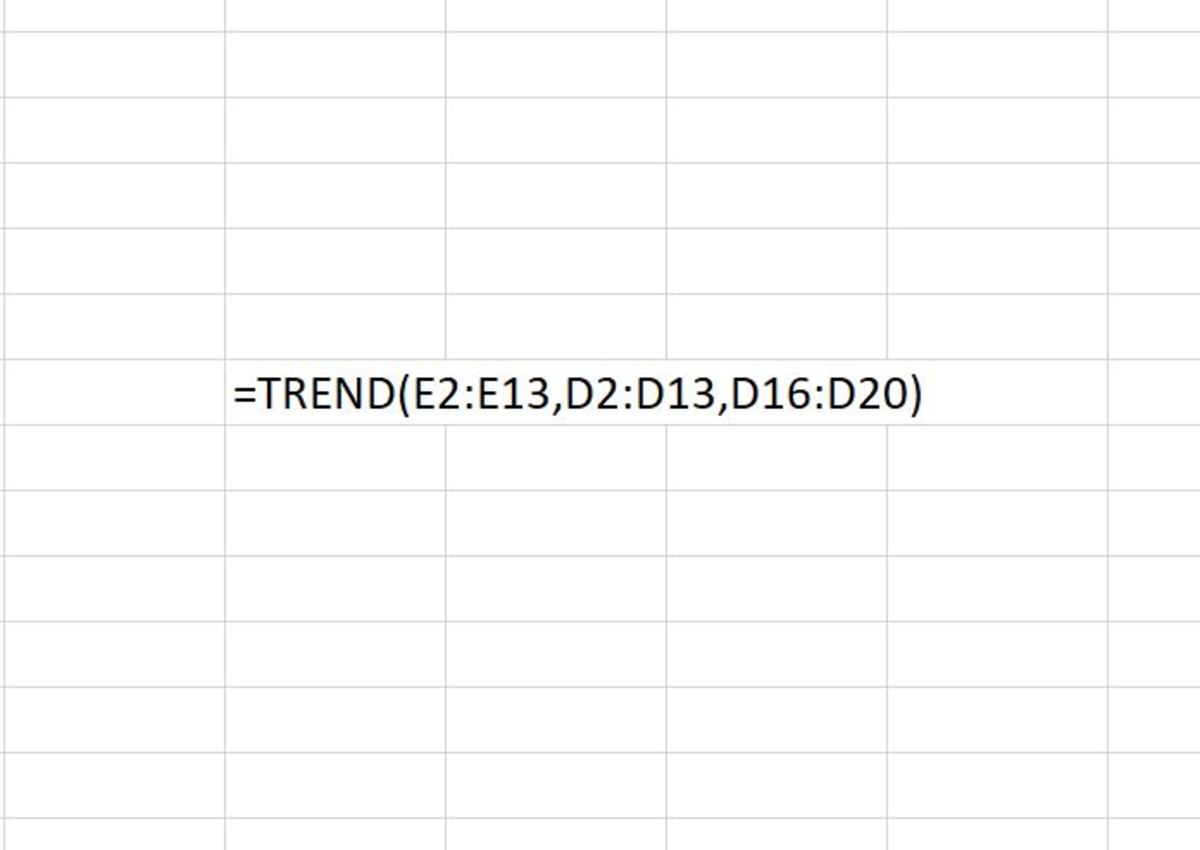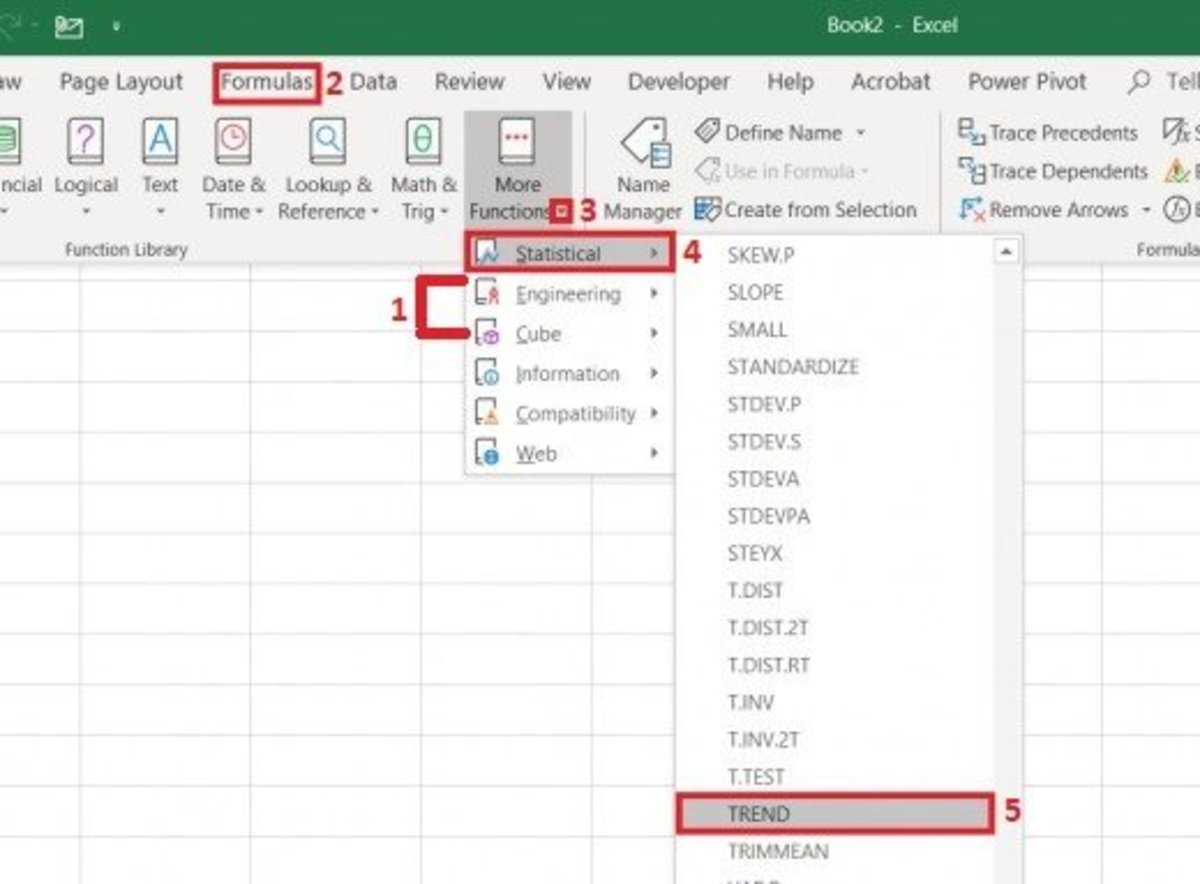Updated date:

# How to Use the TREND Function in Excel

Joshua is a graduate student at the USF. He has interests in business technology, analytics, finance, and lean six sigma.The TREND function uses the least squares method to create predictive values. Essentially the TREND function creates a linear trend, given a linear set of data that is useful for forecasting into the future. Created by Joshua Crowder

## The Purpose of the TREND Function

The TREND function is a statistical function that uses the least squares method to match a data point in a linear trend for which it returns one or more numbers for. Essentially, it predicts the value of y given historical x and y values. The process is explained in more detail in the next section.

The least squared method is used in regression analysis to find the line that best fits. So, this method draws a line to show the relationship between points for a given data set.

## How the TREND Function Works

The TREND function must be inputted into a cell like a formula. To enter the TREND function follow these steps with details about each variable:

1. Click the cell the first predicted value will appear.
2. Type "=TREND(" into the cell without quotation marks.
3. After the open parenthesis, a known array of y values must be added followed by a comma. When selecting this array, keep in mind that when an array of data is selected as a column or row that the variables of variables of x are interpreted separately. These values will be the historical data that you already have.
4. The known array of x values are added followed by a comma. These variables are known in the linear equation y = mx + b. The known x's may be one set or more variables. This depends on how many know y variable you have. For instance, if I was trying to predict my future gas usage based on past days, day 1, day 2, and day 3 may be my know x variables. Be sure that the dimension of the array of known y values is the same as that of the know x values. When more than one variable is used in the function, the known y variables must be a vector (the height and width of the ranges chosen must match).
5. The new x values are added followed by a comma. The new x values are optional. These represent the sequence of the new values. So, looking at the example in the last step, the new x value would be day 4, day 5, day 6 and so on. I recommend using this variable for an more accurate answer because when omitted new x's are assumed to be the same as known x's. You'll notice that your trend data may be underestimated if you plan to predict and this variable is not used. When used, the amount of predicted y's will depend on how many new x variables are selected.
6. Finally, a constant value is selected followed by a closed parenthesis. The constant is also an optional variable. This logical value specifies if b in the equation should equal zero or not. There are 3 different ways to approach this logical setting:
• Set the constant to TRUE to calculate y = mx + b normally.
• Omit a setting to calculate y = mx + b normally.
• Set the constant to FALSE so that b is equal to zero. In this case the m values will be adjusted so y = mx.

The syntax of the TREND function is shown in its entirety below:

=TREND(known_y's, [known_x's], [new_x's], [const])

## Inserting the Trend Function

The TREND function can be added another way by inserting it into a cell from the statistical functions list. By using this method, Excel will walk you through the creation of the function by allow you to enter function variables into fields with explanations for every variable entry. This method is good for this type of function since multiple variables are used and could get mixed up while creating the formula by manually by typing it in a cell. To use this insert type method:

1. Click the the cell where the TREND function needs to appear. This would be in an area where the next trend point would appear.
2. Select the formulas tab in the main tabs section of the Excel Ribbon.
3. Click on the "More Functions" button.
4. Statistical should be chosen along with the TREND selection from the drop down list.

Each step in this process is shown in the illustration below.

## Selecting the Trend FunctionTo insert the TREND function from the formula tab you must first navigate to: Formulas→More Functions→Statistical→TRENDCreated by Joshua Crowder

Next, a functions argument window appears where each of the function's variables can be added. The arrays can either be typed in or selected from the spreadsheet by clicking on the arrow to the right of the array fields. The constant value (TRUE or FALSE) can be typed or a cell reference containing a value can be chosen by clicking on the arrow to the right of that field. Remember that the constant value is optional and not needed for a general trend forecasting. Click the OK button when finished.

## Forecasting Amazon's Annual Sales Trend Example

In this example the Trend function is used to predict annual sales for Amazon Inc. for the next 4 consecutive 10k income statements. This includes the year 2020 through 2024 with trend result based on historical sales data. See the raw data in the table below.

## Amazon Inc. Historical Sales Data

YearAnnual Sales (millions)

2015

\$107,006

2016

\$135,987

2017

\$177,866

2018

\$232,887

2019

\$280,522

Below I have plugged all of these data values into Excel. Again, my goal is to predict the next few years of Amazon's total sales based on their sales history. Instead of inserting from the statistical option, I will be using the insert tool to search for the TREND function.

## Amazon Inc. Sales Data Transferred to Excel

The first thing I'm going to do is select the cell where the first estimated year will show up and then click on the Functions tab in main tabs section. Next, Insert function will be selected. The window in the illustration below will appear. Since the function was recently used I can just click on TREND. Otherwise, I would do a search to find a function that I want to use.

Next, I get to insert all of my ranges. The first would be the array known y values. These are the sales figures that are already known. So, I can select the arrow on right side of the known y's field, then select the array.

It now time to select the known x's. These are going to be the years corresponding to the sales figures that were just selected. Lastly, the unknown x's are selected. The unknown x's are the years that I want to return projected y values for.

After clicking on the OK button, each of the predicted y values are predicted. Remember that only one cell needs to be used to input the function and the rest of the values will fall into place depending on how many unknown x values are selected.

## Spill Error

In the event that you get a spill error as a result, make sure that is no data in the cells where the new y values are supposed to appear. For example, if you are looking for the trends for 2021 to 2025, the formula will be placed where the new y value for 2021 will appear while the other years need to remain blank.

If you still get errors, I would clear those cells or even try to put your data in a few more times.

Microsoft. (n.d.). TREND function. Retrieved January 5, 2020, from https://support.office.com/en-us/article/trend-function-e2f135f0-8827-4096-9873-9a7cf7b51ef1.